# Machine Learning to the Rescue!

MathWorks was recently at RoboCup 2018 in Montreal, Canada. Over the 7 days of this event, we got a lot done. In this post, Sebastian Castro will discuss one of the collaboration efforts he worked on.

# Introduction

One of my favorite things about working with student competitions is the chance to collaborate with teams and organizers. Last year, I got in touch with two professors involved in the RoboCupRescue Simulation League. Allow me to introduce them:

We decided to focus on the Agent Simulation Competition. Participants of this competition need to program a collection of autonomous agents in a simulated disaster scenario; the overall goal being to save as many civilian lives as possible. There are 3 kinds of programmable agents in this challenge:

1. Ambulance team: Pick up injured civilians and take them to shelters
2. Fire brigade: Put out building fires to prevent them from spreading
3. Police force: Clear road blockades to let agents move around the map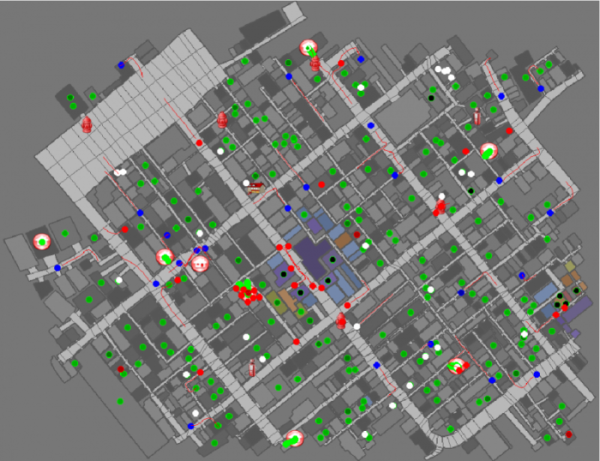Screenshot of a typical RoboCupRescue Agent Simulation

All RoboCup major leagues strive to provide a platform for advancing robotics research. Autonomous behavior and decision-making is increasingly driven by machine learning, and it so happens that MATLAB contains design tools, models, and other functionality for machine learning. As a result, we decided to try integrating MATLAB with the RoboCupRescue Simulation (RCRS) Server using their Agent Development Framework (ADF). Both of these tools are written in Java.

After some time working together, both remotely and at the RoboCup German Open 2018 (Magdeburg, Germany) we came up with a solid proof-of-concept and the idea to deliver a workshop for competition participants. In RoboCup 2018 (Montreal, Canada) we presented a 2-hour “teaser” workshop, had a poster, and won 1st place in the RoboCupRescue Simulation Infrastructure competition! Now, we want to share our work with you.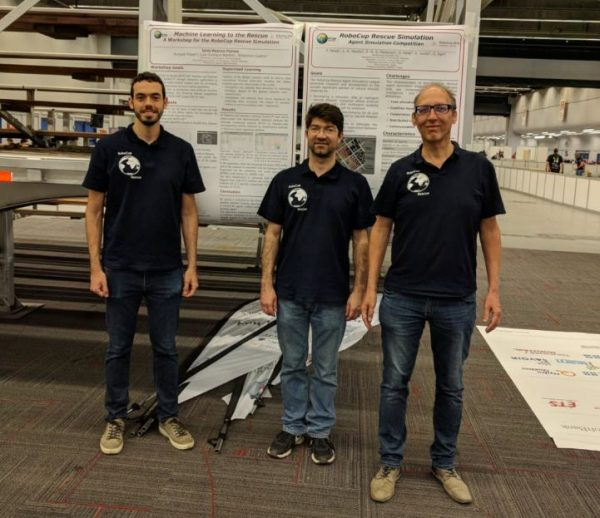Team “Joint Rescue Forces” at RoboCup 2018: Sebastian Castro, Luis Gustavo Nardin, and Arnoud Visser

# Crash Course on Machine Learning

Just as we began our workshop, we will begin with an extremely high-level picture of what Machine Learning is, and how it fits in with its commonly associated buzzwords: Artificial Intelligence and Deep Learning. In the context of robotics, we present the following summaries.

• Artificial Intelligence: Describes a broad set of problems, where an agent has information about the environment and automatically takes action to achieve a goal.
• Machine learning: A subset of artificial intelligence, where an agent uses data to automatically train itself to take action
• Deep learning: A subset of machine learning, which specifically uses neural networks as mathematical models. “Deep” refers to a neural network with many layers, and is a nod to the recent resurfacing of large-scale neural networks due to the computing power available nowadays.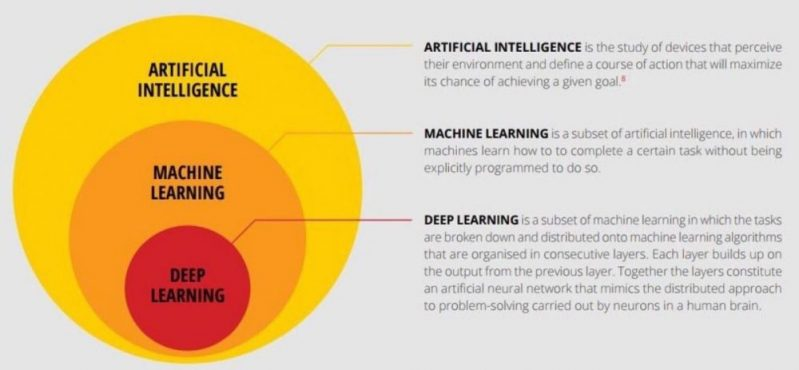AI vs. Machine Learning vs. Deep Learning [Source]

## Types of machine learning problems

Regardless of the machine learning algorithm or model selected (see the next subsection), the same set of tools can be used to solve many types of problems. Below are the four main types of machine learning problems for robotics.

1. Classification

• Labeling input data from a known, finite set of categories
• Examples: Diagnosing disease, identifying types of animals (cats, dogs, horses, etc.)

2. Regression

• Predicting a continuous output from input data
• Examples: Predicting weather (temperature, % rainfall, etc.), calculating actuator forces/torques for robot locomotion

3. Detection

• Locating, counting, and identifying objects of interest in data
• Usually consists of some combination of classification and regression
• Examples: Pedestrian detection, finding key objects and grasping points in cluttered environments

4. Generation

• We can think of this as the “inverse” of classification: synthesizing representative data given a requested category
• Examples: Music/literature generation given a specified style, video game character generation

## Types of machine learning algorithms

Recall that machine learning is defined by the fact that it relies on data. The basic idea is: we provide data to the agent and it forms a generalization, or model, of the problem it needs to solve. A good machine algorithm will be able to accept new, independent data, and correctly solve this problem.

Depending on the format or availability of data, machine learning algorithms can fall into various categories. The main types include:

### 1. Unsupervised Learning

• Finding patterns from unlabeled data
• The agent develops its own insights and we have to make sense of them as best as we can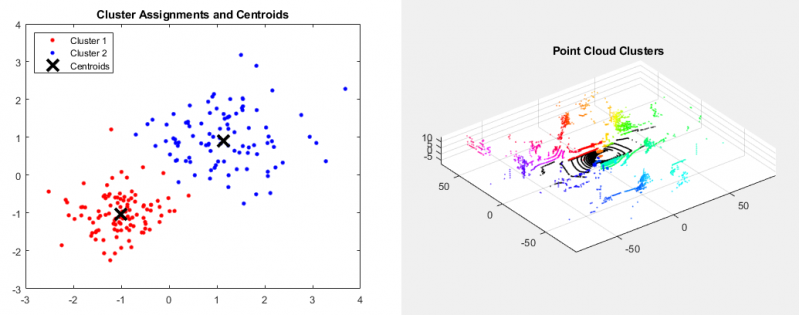Unsupervised Learning Algorithms in MATLAB
[Left] K-Means Clustering for Simple 2D Data | [Right] Euclidean Distance Clustering for Point Cloud Data

### 2. Supervised Learning

• Determining a model, or fitting model parameters, from labeled data
• Since the data is labeled, it is possible for humans to validate models by checking whether the trained model correctly identifies labels on independent test data.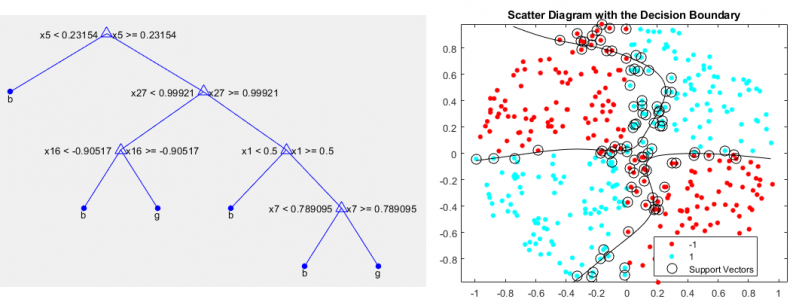Supervised Learning Algorithms in MATLAB
[Left] Decision Tree | [Right] Support Vector Machine (SVM)

### 3. Reinforcement Learning

• Technically, this is a type of supervised learning
• The “label” in this case is a mathematical reward function that the agent needs to maximize
• The agent repeatedly interacts with a physical system (simulated or real-world), evaluates its reward, and learns to maximize it over time.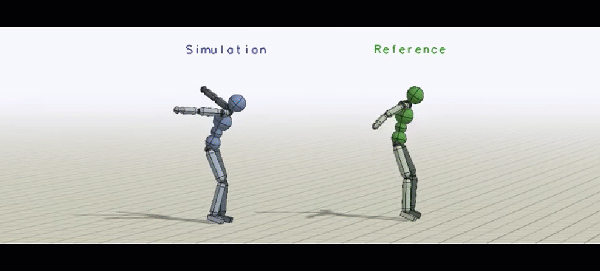DeepMimic: Example-Guided Deep Reinforcement Learning of Physics-Based Character Skills [Source]

NOTE: Deep learning is not a type of algorithm, but rather describes the type of model used by the agent. For example, you might see the term “deep reinforcement learning”. This means that the agent is applying reinforcement learning to tune parameters for its internal deep neural network model.

# AI and Machine Learning for Rescue Agent Simulation

Now that we’ve briefly introduced machine learning, let’s discuss what we did with RoboCupRescue Agent Simulation.

## Path planning

All agents must navigate the roads in a city map to get to their targets. These maps are typically represented as undirected graphs. Graph search is considered an AI problem, but not a machine learning problem since no generalization to new data is required — you simply search over the whole map.

We implemented two alternative solutions for working with graphs: using MATLAB graph objects and graphs from Peter Corke’s Robotics Toolbox.

In both cases, we could generate a graph in MATLAB from the simulator, and then search for the shortest path between any two nodes on the graph using various algorithms. More importantly, once the graph was in MATLAB, each agent could add or remove nodes and edges based on new information (for example, road blockages). These are both important because the simulation:

• Has a precompute step in which the initial map can be calculated and shared among all agents
• Then, at each simulation step, each agent must work with the map independently, including on-the-fly modification and replanning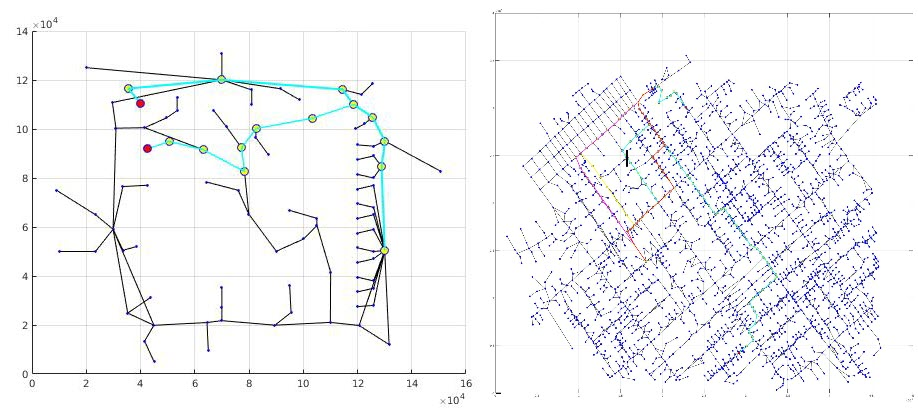[Left] Simple test map with two shortest path solutions
[Right] Map of Kobe, Japan showing shortest path solutions with and without blockades

## Resource allocation

Suppose that your police force consists of 5 police agents. You want to assign different “zones” to them so they can evenly distribute all the tasks that need to be done within the map. How do you position and dispatch your police agents so they can respond to blockades as quickly as possible?

Many teams are already doing this using clustering, which is a type of unsupervised learning. There are many built-in functions for cluster analysis in Statistics and Machine Learning Toolbox.

buildings = importBuildingsData('data/unsupervised/buildings.csv'); % Generated by Import Tool
numMeans = 5;
[indices,centroids] = kmeans([buildings.x buildings.y],numMeans);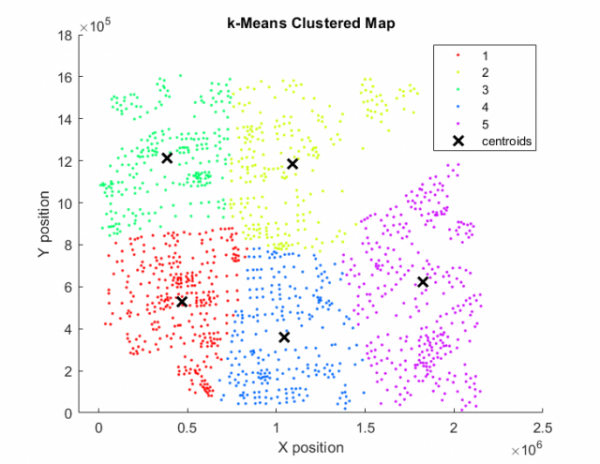Example map showing k-Means Clustering and the corresponding centroids

## Target selection

Now for the final example. Suppose you are an ambulance agent and you are faced with a very uncomfortable, but perhaps realistic, decision: If you have 3 injured civilians to rescue, and some information about them, how do you decide which one to save first?

This is where we can use supervised learning for target selection. We recorded data from previous simulations to a file, which gives us historical information on whether or not a civilian survives being transported to a shelter. The factors, or features, that we logged include:

• Distance from the ambulance
• Health points and injury level when discovered
• The state of the building

An autonomous agent could use this information to make future predictions and prioritize which civilian to rescue, with the intent of maximizing the overall score of the rescue team. For example, the agent could favor rescuing agents predicted to be in a critical state, but still survive the rescue mission.

For the input data, we first used the Import Tool in MATLAB to read spreadsheets and automatically generate a MATLAB function that converts the data to a table. We could then employ techniques such as dimensionality reduction or feature selection to reduce the amount of input data needed to train a model and make a prediction. Ideally, this would lead to a more computationally efficient model, with little to no impact on prediction accuracy.

For the output data, we had to choose the type of machine learning problem to solve. Since our raw output was the number of hit points (HP), from 1 to 10000, we tried the following 3 approaches:

1. Binary classification: Dead (0 HP) vs. Alive (1-10000 HP)
2. Multiclass classification: Dead (0 HP), Critical (1-3000 HP), Injured (3001-7000 HP), and Stable (7001-10000 HP)
3. Regression: Predict the actual HP value from 0 to 10000

The Classification Learner and Regression Learner apps allowed us to try different types of models and find the one with the best accuracy. Then, we could export our trained model and use it to predict on an independent test data set. If the test accuracy was good enough, we could integrate this model into the simulator so each agent could make predictions on new simulation runs.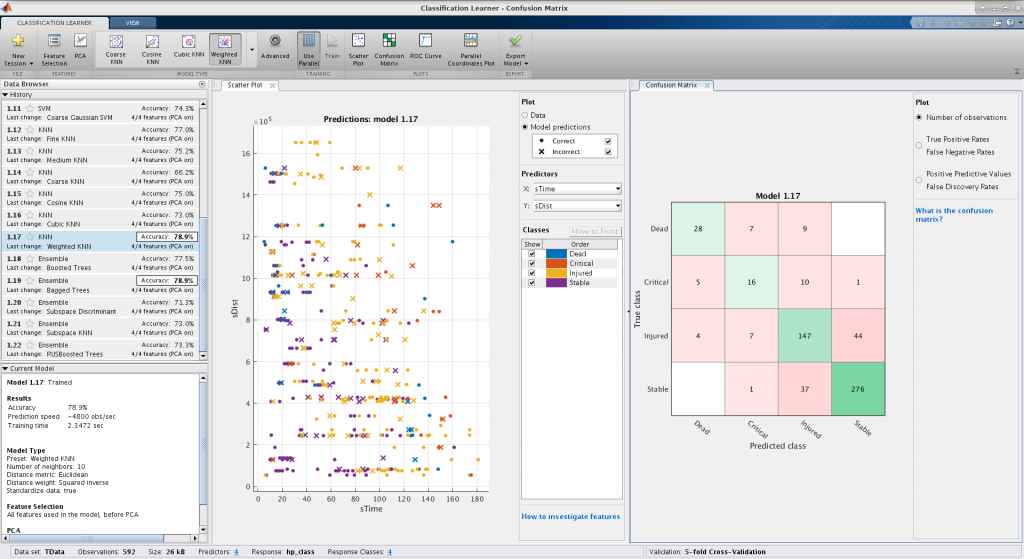Classification Learner app showing multiclass predictions and confusion matrix for our sample data set. We got a maximum accuracy of 78.9% with K-Nearest Neighbors (KNN), which is far better than the 25% we could get from random guessing.

NOTE: We also tried deep learning on this dataset (because, why not?). Since we had a small number of data points and features, and all features were scalar, numeric data, we did not gain much accuracy from the added complexity and nonlinearity of neural networks. However, our repository includes deep learning examples and we encourage you to try them and improve on our work!

# Integrating MATLAB with the Rescue Agent Simulation

Finally, we wanted to discuss integrating the MATLAB based machine learning work with the Java based simulation framework.

## MATLAB Engine API

The MATLAB Engine API for Java lets you call MATLAB code from Java, and pass information between MATLAB and Java, provided that a MATLAB session is currently open on your machine. This was a good first step for prototyping, and we were able to demonstrate this worked with path planning, resource allocation, and target selection tasks from the previous section.

Given the MATLAB Engine API functionality, we were able to explore some design tradeoffs:

• Multiple agents starting separate MATLAB session vs. connecting to a shared MATLAB session
• Evaluating MATLAB commands in the (shared) base workspace vs. calling functions, which have their own data scope
• Calling MATLAB code synchronously (waiting to receive output) vs. asynchronously (executing the code and getting the results later)

By the way: the MATLAB Engine API is available in many other languages as well, including C++ and Python. Refer to the MATLAB documentation for more information.

This approach worked during the precompute step of simulation, but would not scale well to multiple agents because it would require a large number of MATLAB sessions and/or multiple agents trying to access the same shared MATLAB session. Also, there are security/cheating concerns because, after the precompute step of simulation, agents are not allowed to share data with each other.

## Code generation

So, how do we handle multiple agents calling MATLAB code without sharing data or computational resources? The answer: don’t use MATLAB! (and yes, I still work at MathWorks)

Using MATLAB Coder, you can generate portable C/C++ code from the algorithms we described above. This could result in code only, or the code could be automatically compiled into an executable or shared library/object (depending on your operating system).

The best approach we found was generating a shared library and then calling these from each independent agent. No need to have even a single MATLAB instance open, the generated C/C++ code may run faster than the MATLAB code, and there is no need to worry about agents sharing data because each of them will load the library separately.

One small hurdle: Calling C/C++ code from Java requires the Java Native Interface (JNI). Luckily, there are tools available such as Simplified Wrapper and Interface Generator (SWIG) that can do the work for you. Reach out to us if you want to know more.

# Conclusion

In this post, we introduced our own definition of machine learning and some of the common problems and algorithms associated with it. Then, we showed how MATLAB helped us go from design concept to integration with an external software framework… including all the design explorations and tradeoffs we performed along the way.

Our resources are all available online. You can download our code, read our paper, and access our presentation.

Hopefully, we’ve shown you some new things you didn’t know MATLAB could do. If you participate in RoboCup, we hope to see you in an upcoming workshop. Else, we would still like to hear from you in the comments!

|